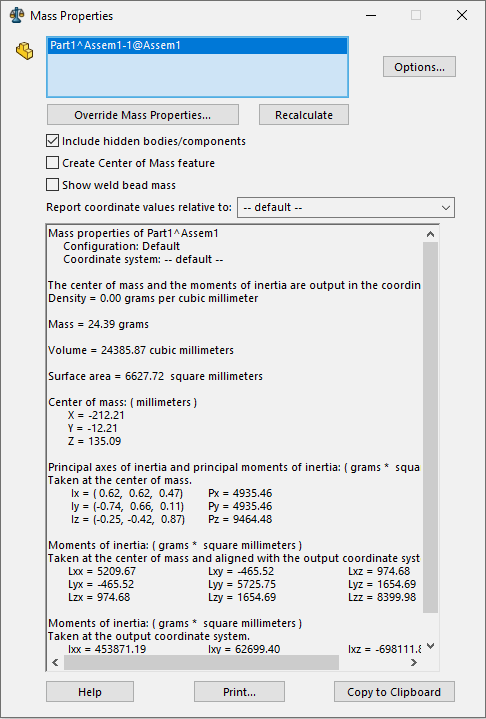# Get center of gravity for the SOLIDWORKS component in assembly

This macro demonstrates 2 approaches to find the component's center of gravity (COG) related ot assembly coordinate system.

Macro will calculate the COG for the selected component.

## Transforming the coordinates

IModelDocExtension::GetMassProperties2 API allows to calculate the mass properties data in the model.

When calculated on the component's model level coordinates need to be transformed into the assembly space using transforms in order to achieve the desired result.

```Dim swApp As SldWorks.SldWorks

Sub main()

Set swApp = Application.SldWorks

Dim swModel As SldWorks.ModelDoc2
Dim swSelMgr As SldWorks.SelectionMgr

Set swModel = swApp.ActiveDoc
Set swSelMgr = swModel.SelectionManager

Dim swComp As SldWorks.Component2

Set swComp = swSelMgr.GetSelectedObjectsComponent3(1, -1)

Dim swCompModel As SldWorks.ModelDoc2
Set swCompModel = swComp.GetModelDoc2

Const ACCURACY_DEFAULT As Integer = 1
Dim status As swMassPropertiesStatus_e

Dim vMassPrps As Variant
vMassPrps = swCompModel.Extension.GetMassProperties2(ACCURACY_DEFAULT, status, False)

Dim dCog(2) As Double

dCog(0) = vMassPrps(0): dCog(1) = vMassPrps(1): dCog(2) = vMassPrps(2)

Dim swMathUtils As SldWorks.MathUtility

Set swMathUtils = swApp.GetMathUtility

Dim swMathPt As SldWorks.MathPoint
Set swMathPt = swMathUtils.CreatePoint(dCog)

Set swMathPt = swMathPt.MultiplyTransform(swComp.Transform2)

Dim vCog As Variant
vCog = swMathPt.ArrayData

Debug.Print "COG: " & vCog(0) & "; " & vCog(1) & "; " & vCog(2)

End Sub
```

## Using IMassProperty interface

IMassProperty interface emulates the functionality of Mass Property feature in SOLIDWORKSMass properties dialog

Similarly to the UI equivalent it is possible to assign bodies (including component bodies) for the calculation scope.

One of the main benefits of this method compared to previous approach is that it is possible to calculate of COG for the lightweight components.

```Dim swApp As SldWorks.SldWorks

Sub main()

Set swApp = Application.SldWorks

Dim swModel As SldWorks.ModelDoc2
Dim swSelMgr As SldWorks.SelectionMgr

Set swModel = swApp.ActiveDoc
Set swSelMgr = swModel.SelectionManager

Dim swComp As SldWorks.Component2

Set swComp = swSelMgr.GetSelectedObjectsComponent3(1, -1)

Dim swMassPrps As SldWorks.MassProperty
Set swMassPrps = swModel.Extension.CreateMassProperty()

Dim vCompBodies As Variant
vCompBodies = swComp.GetBodies3(swBodyType_e.swSolidBody, Empty)

If False <> swMassPrps.AddBodies(vCompBodies) Then

Dim vCog As Variant
vCog = swMassPrps.CenterOfMass

Debug.Print "COG: " & vCog(0) & "; " & vCog(1) & "; " & vCog(2)

Else
Err.Raise vbError, "", "Failed to add bodies for calculation"
End If

End Sub
```